Custom

# Machine Learning for Makers: How to Control an Arduino With WekinatorJuly 01, 2018 by Muhammad Aqib
ShareTrain the machine learning program Wekinator to control LEDs connected to an Arduino UNO.

## Software

 1 Wekinator

## Hardware

 1 Arduino UNO 1 Orange LED 1 White LED 2 220-ohm resistors

In this article, part 6 of a larger machine learning series, you will learn to train Wekinator to control the Arduino. We will train Wekinator to control the LEDs whenever we click on an orange or white box in the processing window.

We will give one input to the Wekinator that will be the value of a variable, and after training the Wekinator, the output will be received by processing, which will further send it to the Arduino where the LEDs will light up.

Catch up on the rest of the Wekinator Machine Learning series here:

### Circuit Diagram

Connect pin 11 of Arduino to the positive lead of the orange LED and connect the negative lead of LED to the ground of Arduino through the 220 ohm resistor. Similarly, connect the positive lead of the white LED to pin 10 of Arduino and the negative lead of the LED to the ground of Arduino through the 220 ohm resistor.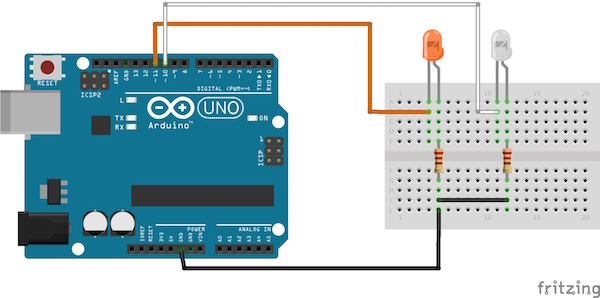### Getting Started with the Program

First, load the code that is provided below for Arduino in the Arduino IDE. Then upload the given code to process in the processing IDE.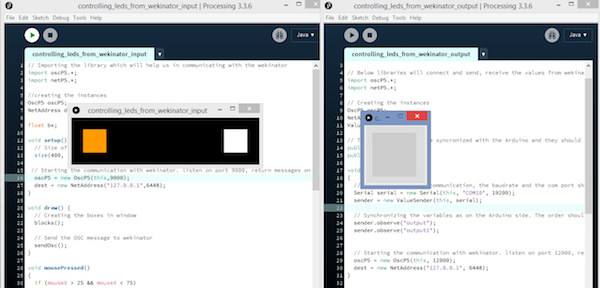After that, open Wekinator and change the inputs to 1 and outputs to 2 and leave the other options as they are.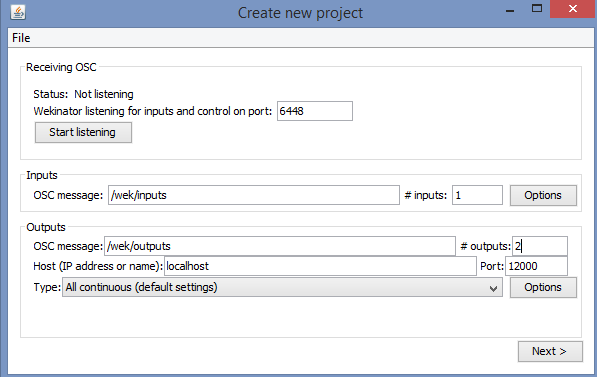Click on â€œnextâ€ and a new window will come up. Now from the input window of processing, click on the orange box and in Wekinator, enter 1 in the output-1 box, and start the recording for half a second.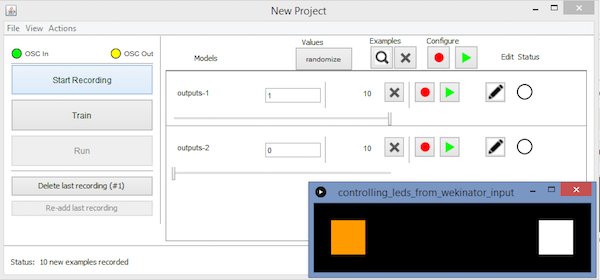Now, click on the white box in processing, and in Wekinator, enter 0 in the output-1 box and enter 1 in the output-2 box and start recording for half a second.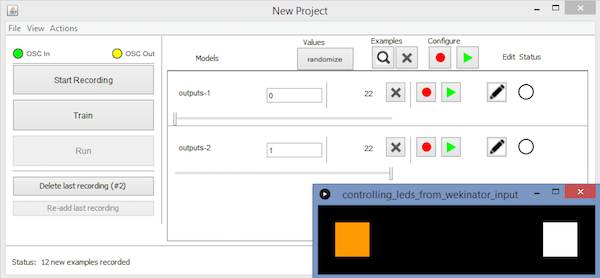Now click on â€œTrainâ€ and then click on â€œRunâ€. Now, when you will click on the orange box, the LED connected to pin 11 will light up, and when you click on the white box, the LED connected to pin 10 of the Arduino will light up.

### Arduino Code

The code is explained with comments.

``````#include <VSync.h>    //Including the library that will help us in receiving and sending the values from processing
Put the number of values to synchronize in the brackets */

/* The below two variables will be synchronized in the processing
and they should be same on both sides. */
int output;
int output1;

// Initializing the pins for led's
int orange_led = 11;
int white_led = 10;

void setup()
{
/* Starting the serial communication because we are communicating with the
Arduino through serial. The baudrate should be same as on the processing side. */
Serial.begin(19200);
pinMode(white_led, OUTPUT);
pinMode(orange_led, OUTPUT);

// Synchronizing the variables with the processing. The variables must be int type.
}

void loop()
{
// Receiving the output from the processing.

// Matching the received output to light up led's
if (output == 1)
{
digitalWrite(orange_led, HIGH);
}
else if (output == 0)
{
digitalWrite(orange_led, LOW);
}

if (output1 == 1)
{
digitalWrite(white_led, HIGH);
}
else if(output1 == 0)
{
digitalWrite(white_led, LOW);
}
}``````

### Processing Code (Input to Wekinator)

``````// Importing the library which will help us in communicating with the wekinator
import oscP5.*;
import netP5.*;

//creating the instances
OscP5 oscP5;

float bx;

void setup() {
// Size of output window
size(400, 100, P3D);

// Starting the communication with wekinator. listen on port 9000, return messages on port 6448
oscP5 = new OscP5(this,9000);
}

void draw() {
// Creating the boxes in window
blocks();

// Send the OSC message to wekinator
sendOsc();
}

void mousePressed()
{
if (mouseX > 25 && mouseX < 75)
{
bx=1;
}

if (mouseX > 325 && mouseX < 375)
{
bx=2;
}

}

void sendOsc() {
OscMessage msg = new OscMessage("/wek/inputs");
oscP5.send(msg, dest);
}

void blocks()
{
background(0);
fill(255, 155, 0);
rect(25, 25, 50, 50);

fill(255, 255, 255);
rect(325, 25, 50, 50);
}``````

### Processing Code (Output From Wekinator)

``````import vsync.*; // Importing the library that will help us in sending and receiving the values from the Arduino
import processing.serial.*;  // Importing the serial library

// Below libraries will connect and send, receive the values from wekinator
import oscP5.*;
import netP5.*;

// Creating the instances
OscP5 oscP5;
ValueSender sender;

// These variables will be syncronized with the Arduino and they should be same on the Arduino side.
public int output;
public int output1;

void setup()
{
// Starting the serial communication, the baudrate and the com port should be same as on the Arduino side.
Serial serial = new Serial(this, "COM10", 19200);
sender = new ValueSender(this, serial);

// Synchronizing the variables as on the Arduino side. The order should be same.
sender.observe("output");
sender.observe("output1");

// Starting the communication with wekinator. listen on port 12000, return messages on port 6448
oscP5 = new OscP5(this, 12000);
}

// Recieve OSC messages from Wekinator
void oscEvent(OscMessage theOscMessage) {
// Receiving the output from wekinator
float value = theOscMessage.get(0).floatValue();  // First output
float value1 = theOscMessage.get(1).floatValue();  // Second output

// Converting the output to int type
output = int(value);
output1 = int(value1);
}
}

void draw()
{
//  Nothing to be drawn for this example
}``````